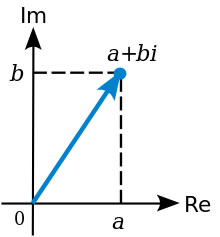# Difference between Real Number and Complex Number

##### Key Difference: A real number is a number that can take any value on the number line. A real number can be any of the rational and irrational numbers. Complex numbers are the numbers that exist in the form of a+ib, where a and b denotes real numbers and i denotes an imaginary part.It is important to understand the concept of number line to learn about real numbers. Number line can be expressed as an actual geometric line where a point is chosen to be the origin. Points that fall in the right side of origin are considered positive numbers, whereas numbers lying in the left side of origin are considered to be negative. A real number refers to any number that can be found on this number line. Therefore, they consist of whole (0,1,3,9,26), rational (6/9, 78.98) and irrational numbers (square root of 3, pi). Infinity does not fall in the category of real numbers. Square root of -1 is also not a real number, and therefore it is referred to as an imaginary number.Complex Numbers are considered to be an extension of the real number system. They have been designed in order to solve the problems, that cannot be solved using real numbers. Complex numbers actually combine real and imaginary number (a+ib), where a and b denotes real numbers, whereas i denotes an imaginary number. By definition, imaginary numbers are those numbers which when squared give a negative result. This statement would not make out a lot of logic as when we calculate the square of a positive number, we get a positive result. Similarly, when a negative number is squared it also provides a positive number. Therefore, imaginary name is given to such numbers. However, unit imaginary number is considered to be the square root of -1.Comparison between Real Number and Complex Number:

 Real Number Complex Number Definition A real number is a number that can take any value on the number line. They can be any of the rational and irrational numbers. Complex Numbers are considered to be an extension of the real number system. Complex numbers actually combine real and imaginary number (a+ib), where a and b denotes real numbers, whereas i denotes an imaginary number. Number line Can be plotted on the number line. Cannot be plotted on the number line. Includes This includes (but is not limited to) positives and negatives, integers and rational numbers, square roots, cube roots , π (pi), etc. This includes numbers like 3 – 2i or 5+√6i, as they can be written as the sum or difference of a real number and an imaginary number. Symbol R C Members All real numbers are also complex numbers with zero for the imaginary part. All imaginary numbers are also complex numbers with zero for the real part.

Image Courtesy: mathpowerblog.wordpress.comom, wikipedia.org